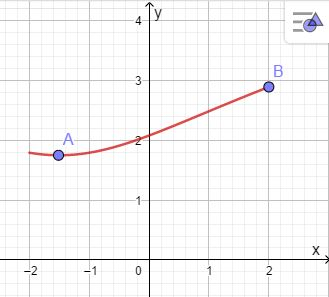# Find the absolute minimum and absolute maximum values of f on the given interval: f(x)=\ln...

## Question:

Find the absolute minimum and absolute maximum values of {eq}f {/eq} on the given interval: {eq}f(x)=\ln (x^2+3x+8), \ [-2,2] {/eq}

## Maximum and Minimum:

We can find the absolute extremes of a function (Maximum and Minimum) at the points where the function reaches its highest increase or decrease.

We have the function

{eq}f(x)=\ln (x^2+3x+8) \\ {/eq}

Differentiating the function

{eq}f'(x)={\frac {2\,x+3}{{x}^{2}+3\,x+8}} \\ {/eq}

{eq}f'(x)=0 {/eq} when {eq}x=-1.5 \\ {/eq}

Graph the functionTherefore,

{eq}f(2)=2.89 \\ f(-2)=1.79 \\ f(-1.5)=1.74 \\ {/eq}

Absolute maximum:

{eq}(2, 2.89) \\ {/eq}

Absolute minimum:

{eq}(-1.5, 1.74) \\ {/eq}